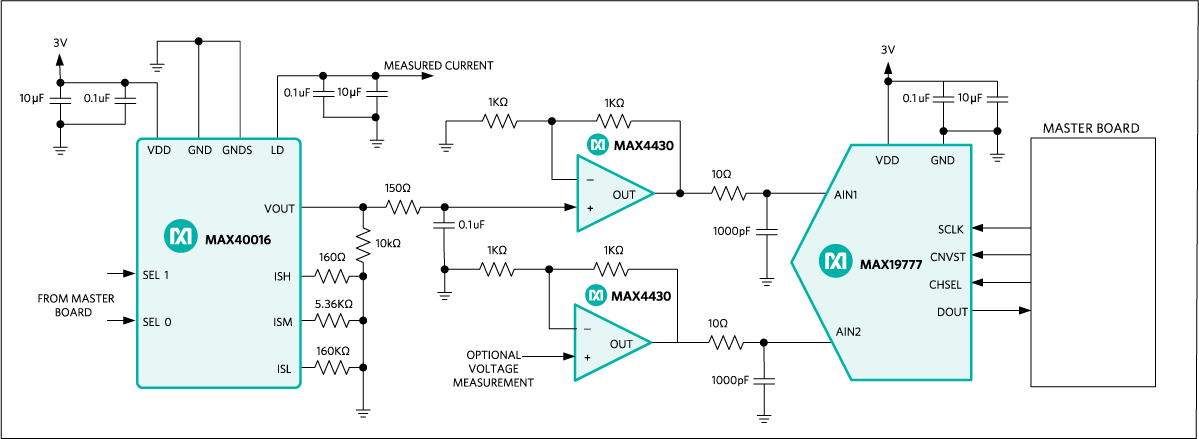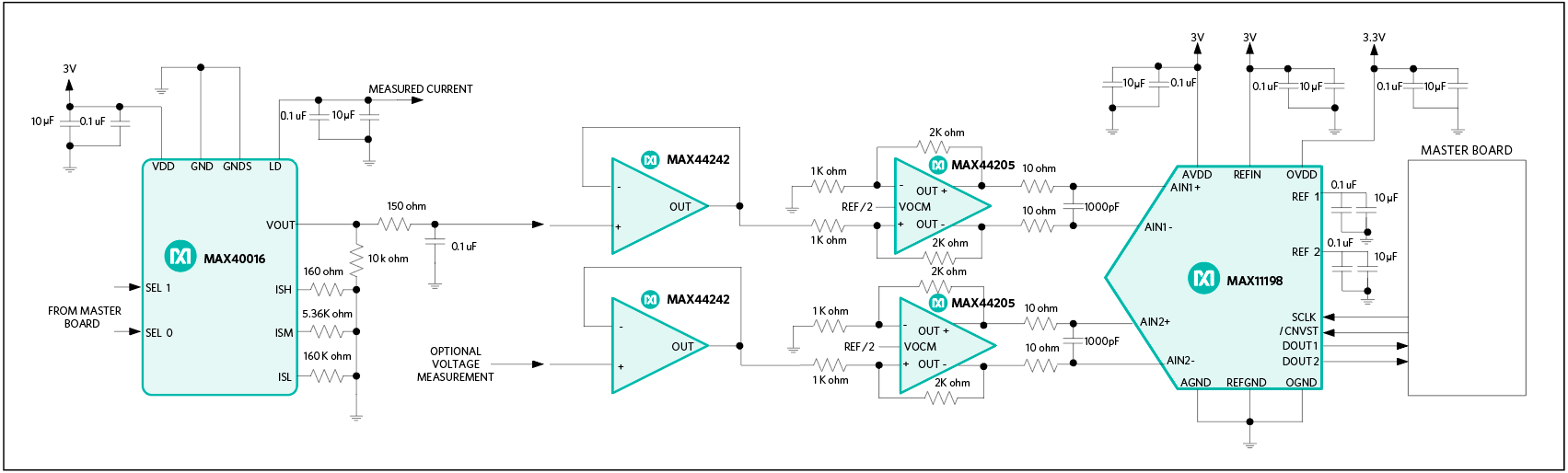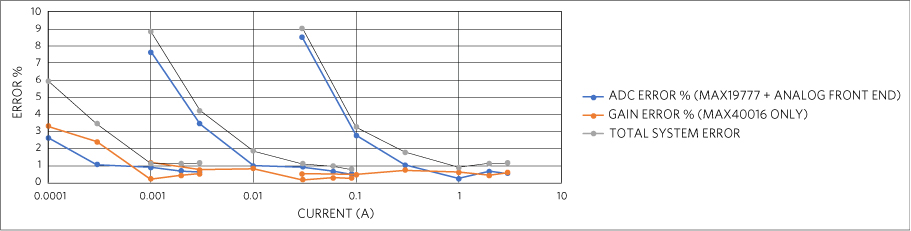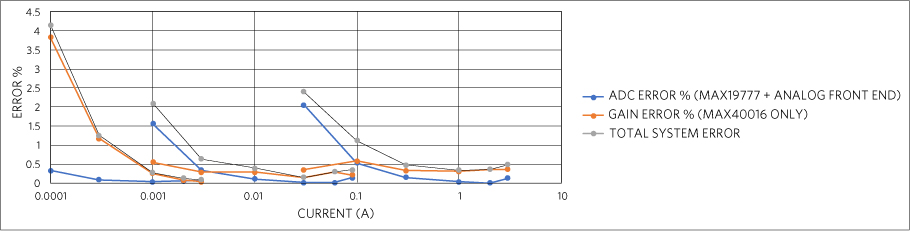# Using Maxim ADCs to Measure the Current on the MAX40016

### 要約

The MAX40016 is a four-decade current-sense amplifier with internal RSENSE. This application note evaluates two Maxim analog-to-digital converters (ADCs) in a MAX40016-based current-sense measurement system to measure the accuracy of the current. The first ADC is the MAX19777, a 12-bit, dual-channel successive approximation register (SAR) analog-to-digital converter; the other is the MAX11198, a 16-bit dual-channel simultaneous-sampling SAR ADC with internal reference

### Introduction

The MAX40016 is a very wide current-sense amplifier with an internal sense element that senses current in a range of 300μA to greater than 3A. This application note evaluates two Maxim analog-to-digital converters (ADCs) in a MAX40016-based current-sense measurement system to measure the accuracy of the current. The ADC measures in volts because the output of the MAX40016 is in voltages. The challenge is to design the analog front end to maximize the potential of the ADC.

This section introduces the two ADCs under evaluation.

#### MAX19777

The MAX19777 is a 16-bit, compact, high-speed, low-power, successive approximation register (SAR) ADC. The supply voltage ranges from 2.2V to 3.3V. Because supply voltage is also the voltage reference, 3V is selected for this design. The output of the MAX40016 is not expected to exceed 1.5V, which is approximately 3A being sensed. An operational amplifier (op amp) stage is placed between the output of the MAX40016 and the input of the MAX19777 to create a gain of 2 that maximizes the full range of the ADC. Figure 1 shows the MAX40016 and MAX19777 in a system.#### MAX11198

The MAX11198 is a dual-channel fully differential SAR ADC with simultaneous sampling at 2Msps. It has a 2.5V internal reference but an external reference can be applied from 2.5V to VAVDD – 0.25V. Again, 3V is selected for this design to maximize the full range of the ADC. A differential amplifier with gain of 2 is placed between the output of the MAX40016 and the input of the MAX11198 that maximizes the full range of the ADC. Figure 2 shows the MAX40016 and MAX11198 in a system.### Measurements

The MAX40016 has three modes for current-range settings: high, middle, and low. Table 1 shows the range tested at each mode in this test.

 Mode Current Range High 30mA to 3A Middle 1mA to 90mA Low 100μA to 3mA

Figure 3 shows the results of the measurements on the MAX40016/MAX19777 system.Figure 4 shows the results of the measurements on the MAX40016/MAX11198 system.At first glance the error percentage might look large, but each mode has some overlap in current ranges. An example is 30mA in high mode versus medium mode. In the MAX40016 and MAX19777 system, at high mode, the total system error percentage is approximately 9%, while in medium mode, the error is approximately 1%. In the MAX40016 and MAX11198 system, at high mode, the total system error percentage is approximately 1.5%, while in medium mode, the total system error percentage is approximately 0.03%. The error percentages improve when more efficient modes are selected.

Through the full current range, the worst error percentage in the MAX40016 and MAX19777 system is approximately 6%, at 100μA. At the MAX40016's minimum specification current range of 300μA, the total system error percentage is approximately 3.5%.

For the MAX40016 and MAX11198 system, the worst error percentage is approximately 0.5%, at 100μA. At the MAX40016's minimum specification current range of 300μA, the total system error percentage is approximately 0.03%.

### Conclusion

Understanding the characteristics of each mode and choosing the right one for a given situation can help the accuracy of the overall system. The MAX11198 is proven to be superior but if a user does not need so much accuracy, the MAX19777 is a viable option. Cost can also be factor in which ADCs makes better sense in your design.